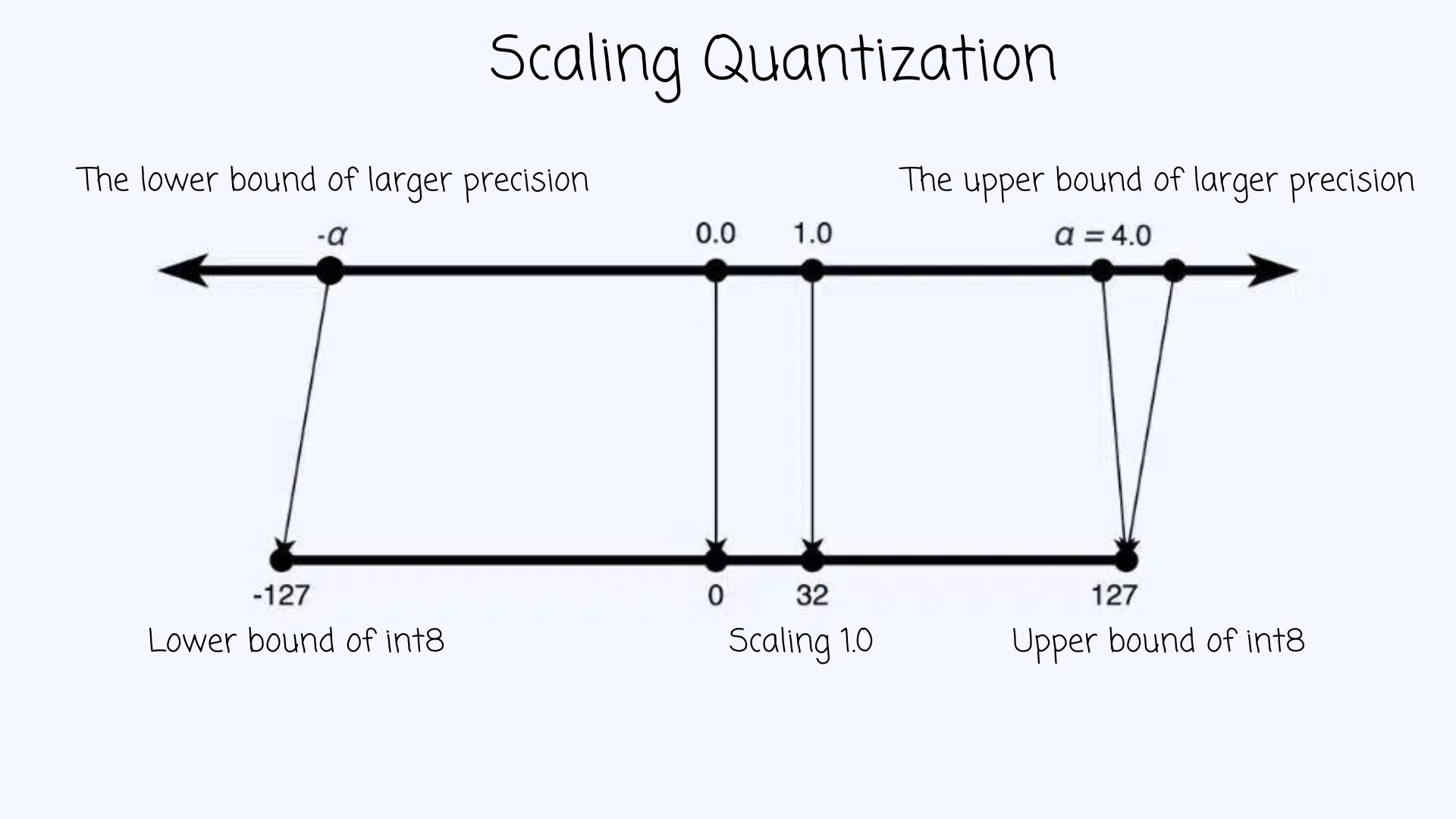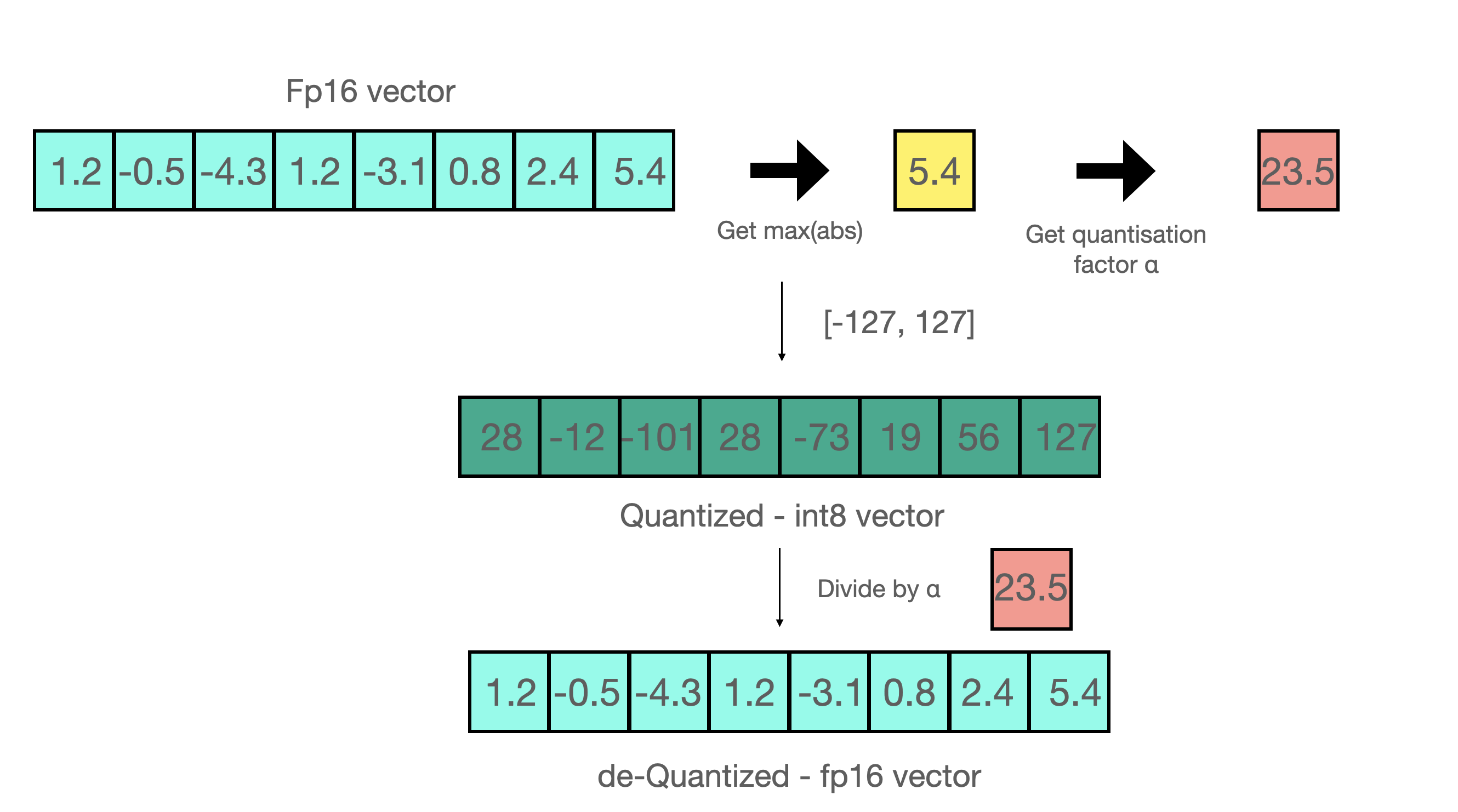# Introduction to Quantization cooked in 🤗 with 💗🧑‍🍳

Community blog post
Published August 25, 2023Quantization is set of techniques to reduce the precision, make the model smaller and training faster in deep learning models. If you didn't understand this sentence, don't worry, you will at the end of this blog post. In this blog post, we will go through..

1. What is precision, why we need quantization and simple quantization example,
2. GPTQ quantization,
3. 4/8-bit (bitsandbytes) quantization.

Let's go!

## Precision

Precision can be defined as the number of significant digits or bits used to represent a number. It's a measure of how precisely a number can be represented or how much detail can be preserved in the representation (in our case, binary representation).

In this blog post, I will not go through how the binary representation used for precision works, but you can check out this intuitive blog post explaining how it works.

We represent numbers in different data types depending on the number itself. Each data type has a range of numbers it can represent. Common data types include:

Data Type Range Precision
FP32 (Single Precision) Approximately ±1.4013 x 10^-45 to ±3.4028 x 10^38 7 decimal digits
FP16 (Half Precision) Approximately ±5.96 x 10^-8 to ±6.55 x 10^4 3-4 decimal digits
FP8 (Custom 8-bit Floating Point) Dynamic Dynamic
Int8 (8-bit Integer) -128 to 127 (signed) or 0 to 255 (unsigned) No decimals

More precise a number needs to be represented, more memory it will occupy. In deep learning, we represent weights with floating point representation (FP32/16/8..). While FP32 representation yields more precision and accuracy, the model size becomes larger and computations during training or inference (depending on the quantization type) become slower. Hence, need to decrease the precision arises, which is also referred to as quantization. It's form of a lossy compression, the more we compress the information, the more we lose performance.

A very simple quantization technique is scaling/projecting the larger range of the bigger quantization type to a smaller scale, e.g. (FP32 to int8). This looks like below 👇For a given range of a data type [-α, α], we can project a given value $ss$ with following formula:

$s=\left({2}^{b-1}\right)-1\mathrm{/}\alpha =127\mathrm{/}\alpha s = \left(2^\left\{b-1\right\}\right)-1/\alpha = 127/\alpha$

However, this method has different disadvantages, e.g. it introduces overhead during training and inference, degrades performance and is sensitive to distribution shifts in data. Though it's very simple, it's heavily used in different mixed precision training methods.

There are two main quantization methods 👇

• Post-training quantization: These methods are focusing on decreasing the precision after the model is trained. Since it's easier to understand, we will mainly go through this in this blog post, though it doesn't perform better than quantization aware training.
• Quantization aware training: This method allows quantizing a model and later fine-tune the model to reduce performance degradation due to quantization, or quantization can take place during training.

The state-of-the-art methods are trying to overcome aforementioned problems. We will now talk about them and how they can be used through tools in 🤗 ecosystem (transformers, TGI, optimum and PEFT).

## GPTQ Quantization

GPTQ is a post-training quantization method to make the model smaller with a calibration dataset. The idea behind GPTQ is very simple: it quantizes each weight by finding a compressed version of that weight, that will yield a minimum mean squared error like below 👇

Given a layer $ll$ with weight matrix ${W}_{l}W_\left\{l\right\}$ and layer input ${X}_{l}X_\left\{l\right\}$, find quantized weight ${\stackrel{^}{W}}_{l}\hat\left\{W\right\}_\left\{l\right\}$:

$\left({{\stackrel{^}{W}}_{l}}^{\ast }=argmi{n}_{\stackrel{^}{{W}_{l}}}\mathrm{\mid }\mathrm{\mid }{W}_{l}X-{\stackrel{^}{W}}_{l}X\mathrm{\mid }{\mathrm{\mid }}_{2}^{2}\right)\left(\left\{\hat\left\{W\right\}_\left\{l\right\}\right\}^\left\{*\right\} = argmin_\left\{\hat\left\{W_\left\{l\right\}\right\}\right\} ||W_\left\{l\right\}X-\hat\left\{W\right\}_\left\{l\right\}X||^\left\{2\right\}_\left\{2\right\}\right)$

In GPTQ, we apply post-quantization for once, and this results in both memory savings and inference speedup (unlike 4/8-bit quantization which we will go through later). AutoGPTQ is a library that enables GPTQ quantization. It is integrated in various libraries in 🤗 ecosystem, to quantize a model, use/serve already quantized model or further fine-tune the model. Let's take a look at how we can do them.

First, install AutoGPTQ:

pip install auto-gptq # for cuda versions other than 11.7, refer to installation guide in above link
pip install transformers optimum peft

• You can run a given GPTQ model on Hugging Face Hub as follows (find them here) 👇
from transformers import AutoModelForCausalLM
model = AutoModelForCausalLM.from_pretrained("TheBloke/Llama-2-7b-Chat-GPTQ", torch_dtype=torch.float16, device_map="auto")


You can quantize any transformers model like below 👇

from transformers import AutoModelForCausalLM, AutoTokenizer, GPTQConfig

tokenizer = AutoTokenizer.from_pretrained(model_id)
quantization_config = GPTQConfig(bits=4, dataset = "c4", tokenizer=tokenizer)

model = AutoModelForCausalLM.from_pretrained(model_id, device_map="auto", quantization_config=quantization_config)

• You can further fine-tune a given GPTQ model using PEFT in this gist.
• You can quantize and serve an already quantized model with GPTQ method using text-generation-inference. It doesn't use AutoGPTQ library under-the-hood. After installing TGI by following the instructions here, you can run a GPTQ model you found on the hub like below 👇
docker run --gpus all --shm-size 1g -p 8080:80 -v $volume:/data ghcr.io/huggingface/text-generation-inference:latest --model-id$model --quantize gptq


To run quantization only with a calibration dataset, simply run

text-generation-server quantize tiiuae/falcon-40b /data/falcon-40b-gptq


You can learn more about the quantization options by running text-generation-server quantize --help.

## 4/8-bit Quantization with bitsandbytes

bitsandbytes is a library used to apply 8-bit and 4-bit quantization to models. It can be used during training for mixed-precision training or before inference to make the model smaller.

8-bit quantization enables multi-billion parameter scale models to fit in smaller hardware without degrading performance. 8bit quantization works as follows 👇

1. Extract the larger values (outliers) columnwise from the input hidden states.
2. Perform the matrix multiplication of the outliers in FP16 and the non-outliers in int8.
3. Scale up the non-outlier results to pull the values back to FP16, and add them to outlier results in FP16.So essentially, we perform the matrix multiplication to save on precision, and then pull the non-outlier results back to FP16 without a lot of difference between non-outlier's initial value and scaled back value. You can see an example below 👇You can install bitsandbytes like below 👇

pip install bitsandbytes


To load a model in 8-bit in transformers, simply run 👇

from transformers import AutoModelForCausalLM, AutoTokenizer
import torch

tokenizer = AutoTokenizer.from_pretrained(name)
encoded_input = tokenizer(text, return_tensors='pt')
output_sequences = model.generate(input_ids=encoded_input['input_ids'].cuda())
print(tokenizer.decode(output_sequences, skip_special_tokens=True))


One caveat of bitsandbytes 8-bit quantization is that the inference speed is slower compared to GPTQ.

4-bit Float (FP4) and 4-bit NormalFloat (NF4) are two data types introduced to use with QLoRA technique, a parameter efficient fine-tuning technique. These data types can also be used to make a pre-trained model smaller without QLoRA. TGI essentially uses these data types for post-training quantization before the inference.

from transformers import AutoModelForCausalLM


In TGI, you can use bitsandbytes quantization techniques by passing --quantize with below values for different quantization methods:
docker run --gpus all --shm-size 1g -p 8080:80 -v $volume:/data ghcr.io/huggingface/text-generation-inference:latest --model-id$model --quantize bitsandbytes